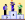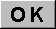Newton's laws of motionQuizz "Physics, Chemistry" - 5 questionstotal = 103 player(s)

Question 1 / 5
 How many laws of motion did Newton make? 1 2 3Question 2 / 5
 Which one of these statements disagree with the first law? an object will accelarate if the resultant force is 0 an object can still be unaccelarated even with given force if there is friction an object can be decelerated (a = -x)Question 3 / 5
 There is a formula in the 2nd law, what is it? a = f/m e = mc2 ep = mghQuestion 4 / 5
 The third law states that for every action there is ... a bigger reaction an equal and opposite reaction a monkey behind the wallQuestion 5 / 5
 these laws are learned in what field? quantum mechanics classical mechanics ecology.
End of the game
Now, let's calculate and register your score.